# Envelope (mathematics)

For the envelope of an oscillating signal, see Envelope (waves).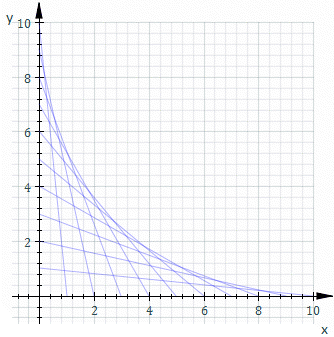Construction of the envelope of a family of curves.

In geometry, an envelope of a family of curves in the plane is a curve that is tangent to each member of the family at some point. Classically, a point on the envelope can be thought of as the intersection of two "adjacent" curves, meaning the limit of intersections of nearby curves. This idea can be generalized to an envelope of surfaces in space, and so on to higher dimensions.

## Envelope of a family of curves

Let each curve Ct in the family be given as the solution of an equation ft(x, y)=0 (see implicit curve), where t is a parameter. Write F(t, x, y)=ft(x, y) and assume F is differentiable.

The envelope of the family Ct is then defined as the set of points (x,y) for whichfor some value of t, whereis the partial derivative of F with respect to t.

Note that if t and u, tu are two values of the parameter then the intersection of the curves Ct and Cu is given byor equivalentlyLetting u→t gives the definition above.

An important special case is when F(t, x, y) is a polynomial in t. This includes, by clearing denominators, the case where F(t, x, y) is a rational function in t. In this case, the definition amounts to t being a double root of F(t, x, y), so the equation of the envelope can be found by setting the discriminant of F to 0 (because the definition demands F=0 at some t and first derivative =0 i.e. it's value 0 and it is min/max at that t).

For example, let Ct be the line whose x and y intercepts are t and 1−t, this is shown in the animation above. The equation of Ct isor, clearing fractions,The equation of the envelope is thenOften when F is not a rational function of the parameter it may be reduced to this case by an appropriate substitution. For example if the family is given by Cθ with an equation of the form u(x, y)cosθ+v(x, y)sinθ=w(x, y), then putting t=eiθ, cosθ=(t+1/t)/2, sinθ=(t-1/t)/2i changes the equation of the curve toorThe equation of the envelope is then given by setting the discriminant to 0:or### Alternative definitions

1. The envelope E1 is the limit of intersections of nearby curves Ct.
2. The envelope E2 is a curve tangent to all of the Ct.
3. The envelope E3 is the boundary of the region filled by the curves Ct.

Then,and, whereis the set of curves given by the first definition at the beginning of this document.

## Examples

### Example 1

These four definitions of the discriminant, E1,E2, and E3, can lead to different sets. Consider for instance the curve y = x3 parametrised by γ : RR2 where γ(t) = (t,t3). The one-parameter family of curves will be given by the tangent lines to γ.

First we calculate the discriminant. The generating function isCalculating the partial derivative Ft = 6t(xt). It follows that either x = t or t = 0. First assume that x = t and t ≠ 0. Substituting into F:and so, assuming that t ≠ 0, it follows that F = Ft = 0 if and only if (x,y) = (t,t3). Next, assuming that t = 0 and substituting into F gives F(0,(x,y)) = y. So, assuming t = 0, it follows that F = Ft = 0 if and only if y = 0. Thus the discriminant is the original curve and its tangent line at γ(0):Next we calculate E1. One curve is given by F(t,(x,y)) = 0 and a nearby curve is given by F(t + ε,(x,y)) where ε is some very small number. The intersection point comes from looking at the limit of F(t,(x,y)) = F(t + ε,(x,y)) as ε tends to zero. Notice that F(t,(x,y)) = F(t + ε,(x,y)) if and only ifIf t ≠ 0 then L has only a single factor of ε. Assuming that t ≠ 0 then the intersection is given bySince t ≠ 0 it follows that x = t. The y value is calculated by knowing that this point must lie on a tangent line to the original curve γ: that F(t,(x,y)) = 0. Substituting and solving gives y = t3. When t = 0, L is divisible by ε2. Assuming that t = 0 then the intersection is given byIt follows that x = 0, and knowing that F(t,(x,y)) = 0 gives y = 0. It follows thatNext we calculate E2. The curve itself is the curve that is tangent to all of its own tangent lines. It follows thatFinally we calculate E3. Every point in the plane has at least one tangent line to γ passing through it, and so region filled by the tangent lines is the whole plane. The boundary E3 is therefore the empty set. Indeed, consider a point in the plane, say (x0,y0). This point lies on a tangent line if and only if there exists a t such thatThis is a cubic in t and as such has at least one real solution. It follows that at least one tangent line to γ must pass through any given point in the plane. If y > x3 and y > 0 then each point (x,y) has exactly one tangent line to γ passing through it. The same is true if y < x3 y < 0. If y < x3 and y > 0 then each point (x,y) has exactly three distinct tangent lines to γ passing through it. The same is true if y > x3 and y < 0. If y = x3 and y ≠ 0 then each point (x,y) has exactly two tangent lines to γ passing through it (this corresponds to the cubic having one ordinary root and one repeated root). The same is true if yx3 and y = 0. If y = x3 and x = 0, i.e., x = y = 0, then this point has a single tangent line to γ passing through it (this corresponds to the cubic having one real root of multiplicity 3). It follows that### Example 2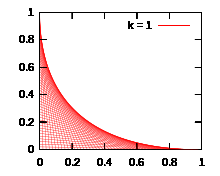This plot gives the envelope of the family of lines connecting points (t,0), (0,k - t), in which k takes 1.

In string art it is common to cross-connect two lines of equally spaced pins. What curve is formed?

For simplicity, set the pins on the x- and y-axes; a non-orthogonal layout is a rotation and scaling away. A general straight-line thread connects the two points (0, kt) and (t, 0), where k is an arbitrary scaling constant, and the family of lines is generated by varying the parameter t. From simple geometry, the equation of this straight line is y = (k  t)x/t + k  t. Rearranging and casting in the form F(x,y,t) = 0 gives:(1)

Now differentiate F(x,y,t) with respect to t and set the result equal to zero, to get(2)

These two equations jointly define the equation of the envelope. From (2) we have t = (y + x + k)/2. Substituting this value of t into (1) and simplifying gives an equation for the envelope in terms of x and y only:This is the familiar implicit conic section form, in this case a parabola. Parabolae remain parabolae under rotation and scaling; thus the string art forms a parabolic arc ("arc" since only a portion of the full parabola is produced). In this case an anticlockwise rotation through 45° gives the orthogonal parabolic equation y = x2/(k√2) + k/(2√2). Note that the final step of eliminating t may not always be possible to do analytically, depending on the form of F(x,y,t).

### Example 3

Let IR be an open interval and let γ : IR2 be a smooth plane curve parametrised by arc length. Consider the one-parameter family of normal lines to γ(I). A line is normal to γ at γ(t) if it passes through γ(t) and is perpendicular to the tangent vector to γ at γ(t). Let T denote the unit tangent vector to γ and let N denote the unit normal vector. Using a dot to denote the dot product, the generating family for the one-parameter family of normal lines is given by F : I × R2R whereClearly (x γ)·T = 0 if and only if x γ is perpendicular to T, or equivalently, if and only if x γ is parallel to N, or equivalently, if and only if x = γ + λN for some λ ∈ R. It follows thatis exactly the normal line to γ at γ(t0). To find the discriminant of F we need to compute its partial derivative with respect to t:where κ is the plane curve curvature of γ. It has been seen that F = 0 if and only if x - γ = λN for some λ ∈ R. Assuming that F = 0 givesAssuming that κ ≠ 0 it follows that λ = 1/κ and soThis is exactly the evolute of the curve γ.

### Example 4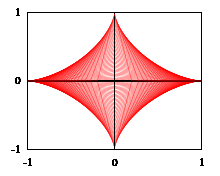An astroid as the envelope of the family of lines connecting points (s,0), (0,t) with s2 + t2 = 1

The following example shows that in some cases the envelope of a family of curves may be seen as the topologic boundary of a union of sets, whose boundaries are the curves of the envelope. Forandconsider the (open) right triangle in a Cartesian plane with vertices,andFix an exponent, and consider the union of all the trianglessubjected to the constraint, that is the open setTo write a Cartesian representation for, start with any,satisfyingand any. The Hölder inequality inwith respect to the conjugated exponentsandgives:,

with equality if and only if. In terms of a union of sets the latter inequality reads: the pointbelongs to the set, that is, it belongs to somewith, if and only if it satisfiesMoreover, the boundary inof the setis the envelope of the corresponding family of line segments(that is, the hypotenuses of the triangles), and has Cartesian equationNotice that, in particular, the valuegives the arc of parabola of the example 1, and the value(meaning that all hypotenuses are unit length segments) gives the astroid.

### Example 5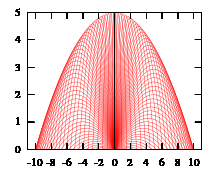The orbits' envelope of the projectiles (with constant initial speed) is a concave parabola. The initial speed is 10 m/s. We take g = 10 m/s2.

We consider the following example of envelope in motion. Suppose at initial height 0, one casts a projectile into the air with constant initial velocity v but different elevation angles θ. Let x be the horizontal axis in the motion surface, and let y denote the vertical axis. Then the motion gives the following differential dynamical system:which satisfies four initial conditions:Here t denotes motion time, θ is elevation angle, g denotes gravitational acceleration, and v is the constant initial speed (not velocity). The solution of the above system can take an implicit form:To find its envelope equation, one may compute the desired derivative:By eliminating θ, one may reach the following envelope equation:Clearly the resulted envelope is also a concave parabola.

## Envelope of a family of surfaces

A one-parameter family of surfaces in three-dimensional Euclidean space is given by a set of equationsdepending on a real parameter a. For example the tangent planes to a surface along a curve in the surface form such a family.

Two surfaces corresponding to different values a and a' intersect in a common curve defined byIn the limit as a' approaches a, this curve tends to a curve contained in the surface at aThis curve is called the characteristic of the family at a. As a varies the locus of these characteristic curves defines a surface called the envelope of the family of surfaces.

The envelope of a family of surfaces is tangent to each surface in the family along the characteristic curve in that surface.

## Generalisations

The idea of an envelope of a family of smooth submanifolds follows naturally. In general, if we have a family of submanifolds with codimension c then we need to have at least a c-parameter family of such submanifolds. For example: a one-parameter family of curves in three-space (c = 2) does not, generically, have an envelope.

## Applications

### Ordinary differential equations

Envelopes are connected to the study of ordinary differential equations (ODEs), and in particular singular solutions of ODEs. Consider, for example, the one-parameter family of tangent lines to the parabola y = x2. These are given by the generating family F(t,(x,y)) = t2 – 2tx + y. The zero level set F(t0,(x,y)) = 0 gives the equation of the tangent line to the parabola at the point (t0,t02). The equation t2 – 2tx + y = 0 can always be solved for y as a function of x and so, considerSubstitutinggives the ODENot surprisingly y = 2tx  t2 are all solutions to this ODE. However, the envelope of this one-parameter family of lines, which is the parabola y = x2, is also a solution to this ODE. Another famous example is Clairaut's equation.

### Partial differential equations

Envelopes can be used to construct more complicated solutions of first order partial differential equations (PDEs) from simpler ones. Let F(x,u,Du) = 0 be a first order PDE, where x is a variable with values in an open set Ω  Rn, u is an unknown real-valued function, Du is the gradient of u, and F is a continuously differentiable function that is regular in Du. Suppose that u(x;a) is an m-parameter family of solutions: that is, for each fixed a  A  Rm, u(x;a) is a solution of the differential equation. A new solution of the differential equation can be constructed by first solving (if possible)for a = φ(x) as a function of x. The envelope of the family of functions {u(·,a)}aA is defined byand also solves the differential equation (provided that it exists as a continuously differentiable function).

Geometrically, the graph of v(x) is everywhere tangent to the graph of some member of the family u(x;a). Since the differential equation is first order, it only puts a condition on the tangent plane to the graph, so that any function everywhere tangent to a solution must also be a solution. The same idea underlies the solution of a first order equation as an integral of the Monge cone. The Monge cone is a cone field in the Rn+1 of the (x,u) variables cut out by the envelope of the tangent spaces to the first order PDE at each point. A solution of the PDE is then an envelope of the cone field.

In Riemannian geometry, if a smooth family of geodesics through a point P in a Riemannian manifold has an envelope, then P has a conjugate point where any geodesic of the family intersects the envelope. The same is true more generally in the calculus of variations: if a family of extremals to a functional through a given point P has an envelope, then a point where an extremal intersects the envelope is a conjugate point to P.

### Caustics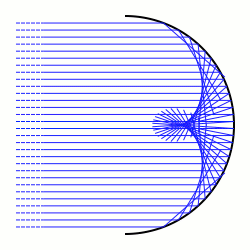Reflective caustic generated from a circle and parallel rays

In geometrical optics, a caustic is the envelope of a family of light rays. In this picture there is an arc of a circle. The light rays (shown in blue) are coming from a source at infinity, and so arrive parallel. When they hit the circular arc the light rays are scattered in different directions according to the law of reflection. When a light ray hits the arc at a point the light will be reflected as though it had been reflected by the arc's tangent line at that point. The reflected light rays give a one-parameter family of lines in the plane. The envelope of these lines is the reflective caustic. A reflective caustic will generically consist of smooth points and ordinary cusp points.

From the point of view of the calculus of variations, Fermat's principle (in its modern form) implies that light rays are the extremals for the length functionalamong smooth curves γ on [a,b] with fixed endpoints γ(a) and γ(b). The caustic determined by a given point P (in the image the point is at infinity) is the set of conjugate points to P.

### Huygens's principle

Light may pass through anisotropic inhomogeneous media at different rates depending on the direction and starting position of a light ray. The boundary of the set of points to which light can travel from a given point q after a time t is known as the wave front after time t, denoted here by Φq(t). It consists of precisely the points that can be reached from q in time t by travelling at the speed of light. Huygens's principle asserts that the wave front set Φq0(s + t) is the envelope of the family of wave fronts Φq(s) for q  Φq0(t). More generally, the point q0 could be replaced by any curve, surface or closed set in space.

## References

1. Bruce, J. W.; Giblin, P. J. (1984), Curves and Singularities, Cambridge University Press, ISBN 0-521-42999-4
2. Eisenhart, Luther P. (2008), A Treatise on the Differential Geometry of Curves and Surfaces, Schwarz Press, ISBN 1-4437-3160-9
3. Forsyth, Andrew Russell (1959), Theory of differential equations, Six volumes bound as three, New York: Dover Publications, MR 0123757, §§100-106.
4. Evans, Lawrence C. (1998), Partial differential equations, Providence, R.I.: American Mathematical Society, ISBN 978-0-8218-0772-9.
5. John, Fritz (1991), Partial differential equations (4th ed.), Springer, ISBN 978-0-387-90609-6.
6. Born, Max (October 1999), Principle of Optics, Cambridge University Press, ISBN 978-0-521-64222-4, Appendix I: The calculus of variations.
7. Arnold, V. I. (1997), Mathematical Methods of Classical Mechanics, 2nd ed., Berlin, New York: Springer-Verlag, ISBN 978-0-387-96890-2, §46.